• Matplotlib是一个Python工具箱，用于科学计算的数据可视化。借助它，Python可以绘制如Matlab和Octave多种多样的数据...下面这篇文章主要介绍了python使用matplotlib如何绘制折线图的方法教程，需要的朋友可以参考借鉴。
• matplotlib画折线图

千次阅读 2018-12-16 21:16:46
matplotlib画折线图 假设一天中每隔两个小时(range(2,26,2))的气温(℃)分别是[15,13,14.5,17,20,25,26,26,27,22,18,15] 代码如下： # 导入pyplot from matplotlib import pyplot as plt # 数据在X轴的位置，是一...

matplotlib画折线图

• 假设一天中每隔两个小时(range(2,26,2))的气温(℃)分别是[15,13,14.5,17,20,25,26,26,27,22,18,15]

代码如下：

# 导入pyplot
from matplotlib import pyplot as plt

# 数据在X轴的位置，是一个可迭代的对象
x = range(2, 26, 2)
# 数据在Y轴的位置，是一个可迭代的对象
# X轴与Y轴的数据个数必须一样，否则会报错
# X轴和Y轴的数据一起组成了所有要绘制出的坐标
# 分别是（2,15）,(4,13)...
y = [15,13,14.5,17,20,25,26,26,27,22,18,15]
# 传入x,y，通过pyplot绘制出折线图
plt.plot(x, y)
# 展示图形
plt.show()

结果如下图所示：• 从图中可以看出，还存在一下几个问题：

1. 设置图片大小（想要一个高清大图）

• 使用figure中的参数figsize调整大小
from matplotlib import pyplot as plt

# figure图形图标的意思，在这里就是指我们画的图
# 通过实例化一个figure并且传递参数
# 在图像模糊的时候可以传入dpi参数，让图片更加清晰
fig = plt.figure(figsize=(20, 8), dpi=80)

x = range(2, 26, 2)
y = [15,13,14.5,17,20,25,26,26,27,22,18,15]
plt.plot(x, y)
plt.show()2. 保存到本地

• 使用savefig，plt.savefig(“保存路径文件名”)
from matplotlib import pyplot as plt
fig = plt.figure(figsize=(20, 8), dpi=80)
x = range(2, 26, 2)
y = [15,13,14.5,17,20,25,26,26,27,22,18,15]
plt.plot(x, y)
# 保存图片
plt.savefig("./t1.png")

3. 描述信息，比如x轴和y轴分别表示什么，这个图表示什么

• x轴的描述信息使用：plt.xlabel()
• y轴的描述信息使用：plt.ylabel()
• 图的描述信息使用：plt.title()
4. 设置x或者y轴上数字和字符串对应

• x轴使用：plt.xticks(“数字”，“字符串”)
• y轴使用：plt.yticks(“数字”，“字符串”)
5. 显示中文（matplotlib不支持中文显示）

• 从matplotlib中导入font_manager的包
• 查看系统中支持的中文字体
• my_font=font_manager.fontProperties(fname=“字体路径”)
• 在需要使用中文显示的地方加入：fontproperties=my_font
6. 线条的样式（比如颜色，透明度等）

• 在绘制的时候指定即可，即在plt.plot()中
• 线条风格：linestyle=’–’
字符线条风格
-实线
虚线
-.点划线
:点虚线
• 线条粗细：linewidth=5
• 透明度：alpha=0.5
• 设置线条颜色：color=‘r’,也可以使用16进制表示颜色，也可以使用英文。
字符颜色
r红色
g绿色
b蓝色
w白色
7. 在一张图中绘制多个折线

• 需要多少个线，就plot多少次
8. 设置图例

• 绘制多个折线之后，无法看出哪条折线代表什么意思，因此需要使用图例
• 首先在plt.plot()中添加label,区别不同的折线
• plt.legend(prop=my_font,loc="'best")
• 通过prop指定图例的字体
• 通过loc指定图例的位置，默认右上角
9. 显示网格

• plt.grid()
• 例题：如果列表a表示10点到12点的每一分钟的气温,如何绘制折线图观察每分钟气温的变化情况?
y = [random.randint(20,35) for i in range(120)]

from matplotlib import pyplot as plt
import random
from matplotlib import font_manager
# 设置中文显示，fname表示字体的路径。
# 在需要显示中文的地方加上fontproperties = my_font
my_font = font_manager.FontProperties(fname="C:\Windows\Fonts\msyh.ttc")

y = [random.randint(20,35) for i in range(120)]
x = range(0, 120)

fig = plt.figure(figsize=(20,8),dpi=80)
_x = list(x)
# _xtick_labels = ["hello,{}".format(i) for i in _x]
_xtick_labels = ["10点{}分".format(i) for i in range(60) ]
_xtick_labels += ["11点{}分".format(i) for i in range(60)]
# 让列表x中的数据和_xtick_labels上的数据都传入，最终会在x轴上一一对应显示
# 两组数据的长度必须一样，否则不能完全覆盖整个轴
# [::5]使用列表切片，每隔5个选一个数据进行展示
# rotation=45表示旋转45°，这样字符串之间不会覆盖
plt.xticks(_x[::5], _xtick_labels[::5],rotation = 45, fontproperties = my_font)

plt.xlabel("时间", fontproperties = my_font)
plt.ylabel("温度(ºC)",fontproperties = my_font)
plt.title("10点到12点每分钟温度的变化情况", fontproperties = my_font)
plt.plot(x,y)
plt.show()

结果展示：• 例题：假设大家在30岁的时候,根据自己的实际情况,统计出来了从11岁到30岁每年交的女(男)朋友的数量如列表a,请绘制出该数据的折线图,以便分析自己每年交女(男)朋友的数量走势
y = [1,0,1,1,2,4,3,2,3,4,4,5,6,5,4,3,3,1,1,1]
要求:
y轴表示个数
x轴表示岁数,比如11岁,12岁等
from matplotlib import pyplot as plt
from matplotlib import font_manager

my_font = font_manager.FontProperties(fname="C:\Windows\Fonts\msyh.ttc")

x = range(11, 31)
y = [1,0,1,1,2,4,3,2,3,4,4,5,6,5,4,3,3,1,1,1]

fig = plt.figure(figsize=(20,8), dpi=80)

_x = x
_xtick_labels = ["{}岁".format(i) for i in _x]
plt.xticks(x, _xtick_labels, fontproperties = my_font)

plt.title("11岁到30之间每年交的女朋友数量",fontproperties = my_font)

# 绘制网格
plt.grid(alpha = 0.1)

plt.plot(x, y)
plt.show()

结果展示：• 例题：假设大家在30岁的时候,根据自己的实际情况,统计出来了你和你同桌各自从11岁到30岁每年交的女(男)朋友的数量如列表a和b,请在一个图中绘制出该数据的折线图,以便比较自己和同桌20年间的差异,同时分析每年交女(男)朋友的数量走势
y_1 = [1,0,1,1,2,4,3,2,3,4,4,5,6,5,4,3,3,1,1,1]
y_2 = [1,0,3,1,2,2,3,3,2,1 ,2,1,1,1,1,1,1,1,1,1]
要求:
y轴表示个数
x轴表示岁数,比如11岁,12岁等
from matplotlib import pyplot as plt
from matplotlib import font_manager

my_font = font_manager.FontProperties(fname="C:\Windows\Fonts\msyh.ttc")

x = range(11, 31)
y_1 = [1,0,1,1,2,4,3,2,3,4,4,5,6,5,4,3,3,1,1,1]
y_2 = [1,0,3,1,2,2,3,3,2,1 ,2,1,1,1,1,1,1,1,1,1]

fig = plt.figure(figsize=(20,8), dpi=80)
plt.plot(x, y_1,label="自己")
plt.plot(x, y_2, label="同桌")
_x = x
_xtick_labels = ["{}岁".format(i) for i in _x]
plt.xticks(x, _xtick_labels, fontproperties = my_font)

plt.title("11岁到30之间每年交的女朋友数量",fontproperties = my_font)

plt.legend(prop = my_font,loc = "best")

# 绘制网格
plt.grid(alpha = 0.5)

plt.show()

结果展示：展开全文matplotlib 折线图 数据分析
• #numpy,matplotlib,pandas为数据分析三剑客。 import numpy as np import pandas as pd import matplotlib.pyplot as plt from matplotlib.ticker ...#使用numpy产生数据 x = np.arange(-5, 5 , 0.1) ‘’’ np.ar...
#numpy,matplotlib,pandas为数据分析三剑客。
import numpy as np
import pandas as pd
import matplotlib.pyplot as plt
from matplotlib.ticker import MultipleLocator

#使用numpy产生数据
x = np.arange(-5, 5 , 0.1)
'''
np.arange()函数返回一个有终点和起点的固定步长的数列，里面可以设置1-3个参数，依次是起点数，步长和终点数。
一个参数时，参数值为终点，起点取默认值0，步长取默认值1。
两个参数时，第一个参数为起点，第二个参数为终点，步长取默认值1。
三个参数时，第一个参数为起点，第二个参数为终点，第三个参数为步长。其中步长支持小数。
'''
y = x*3

#创建窗口和子图
#方法一：先创建窗口，再创建子图（空白处绘制，子图填满窗口）
fig = plt.figure(num = 1, figsize=(15, 8), dpi=80) #开启一个窗口，设置宽高和dpi。
'''
figure()函数说明
figure(num=None, figsize=None, dpi=None, facecolor=None, edgecolor=None, frameon=True)
num:图像编号或名称，数字为编号 ，字符串为名称
figsize:指定figure的宽和高，单位为英寸；
dpi参数指定绘图对象的分辨率，即每英寸多少个像素，缺省值（默认值）为80。1英寸等于2.5cm,A4纸是 21*30cm的纸张
facecolor:背景颜色
edgecolor:边框颜色
frameon:是否显示边框

'''
print(fig, ax1, ax2) #打印窗口和子图参数'''

#设置子图的基本元素
ax1.set_title('python_drawing') #设置标题
ax1.set_xlabel('x_name') #设置X轴名称
ax1.set_ylabel('y_name') #设置Y轴名称
plt.axis([-5,5,-16,16]) #设置横、纵坐标的范围
'''
#上面axis（）函数的结果可以通过以下两个函数分解实现
ax1.set_xlim(-6,6)
ax1.set_ylim(-10,10)
'''
#定义横、纵向主刻度标签的刻度差，就是隔几个刻度才显示一个标签文本。
xmajorLocator = MultipleLocator(2)
ymajorLocator = MultipleLocator(2)
#X轴、Y轴应用定义的横、纵向主刻度，如果不应用将采用默认刻度。
ax1.xaxis.set_major_locator(xmajorLocator)
ax1.yaxis.set_major_locator(ymajorLocator)
#X轴、Y轴的网格应用定义的横、纵向主刻度。
ax1.xaxis.grid(True, which='major') #两个参数，为Flase时不显示网格，括号为空为默认值（显示网格，采用主刻度）。
ax1.yaxis.grid(True, which='major')

'''
#删除X轴、Y轴刻度
ax1.set_xticks([])
ax1.set_yticks([])
# 自由设置X轴、Y轴刻度
ax1.set_xticks([-5,-2,-1,1,3,5])
ax1.set_yticks([-1,-3,-7,-9])
'''

'''#设置刻度的显示文本,设置刻度时前后要各多一个，rotation旋转角度，fontsize字体大小（small/medium/large）
ax1.set_xticklabels(labels=['x0', 'x1', 'x2', 'x3', 'x4', 'x5', 'x6', 'x7', 'x8'], rotation = -45, fontsize = 'small')
ax1.set_yticklabels(labels=['y1', 'y2'], rotation = 0, fontsize = 'medium')
'''

#设置线条参数
plot1 = ax1.plot(x,y, linestyle='',marker = 's',color='g',label = 'legend8',alpha=0.5,linewidth=2)
#alpha透明度，label为图例中线条名称，linewidth线条宽度。
'''
plt.plot()函数说明：
一、plt.plot(x,y,format_string,**kwargs)

x,y:x轴数据，y轴数据

format_string:控制曲线的格式字串,有线条风格、线条标记、线条颜色三部分。
线条风格，linestyle（或ls）=’-’ 当参数为空时是点图
线条标记，marker = ‘o’
线条颜色，color=’g’

以上三个参数的可选值
线条风格（linestyle）
‘-‘                         实线
‘:’                         虚线
‘–’                         破折线
‘None’ ’’               什么都不画
‘-.’                        点划线
线条标记（marker）
‘.’                 点
‘D’                 菱形
‘s’                 正方形
‘h’                 六边形1
‘*’                 星号
‘H’                 六边形2
‘d’                 小菱形
‘_’                 水平线
‘v’                 一角朝下的三角形
‘8’                 八边形
‘<’                 一角朝左的三角形
‘p’                 五边形
‘>’                 一角朝右的三角形
‘,’                 像素
‘^’                 一角朝上的三角形
‘+’                 加号
‘\  ‘               竖线
‘None’,’’         无
‘x’                 X
线条颜色（color）
b               蓝色
g               绿色
r               红色
y               黄色
c               青色
k               黑色
m               洋红色
w               白色

关于*kwargs：
有时候，函数的参数里会有(*args, **kwargs)，都是可变参数，*args表示无名参数，是一个元组
**kwargs是键值参数，相当于一个字典
比如你输入参数为：(1,2,3,4,k,a=1,b=2,c=3)，*args=(1,2,3,4,k)，**kwargs={'a':'1,'b':2,'c':3}
如果同时使用这两个参数，*args要在**kwargs之前
本例子中，linestyle='',marker = 's',color='g', alpha=0.5,linewidth=2等键值对都是**kwargs
'''
#添加数字标签
for a,b in zip(x,y):
plt.text(a, b+0.05, '%.0f' % b, ha='center', va= 'bottom',fontsize=12)
'''
首先，前边设置的x、y值其实就代表了不同柱子在图形中的位置（坐标），通过for循环找到每一个x、y值的相应坐标——a、b，
再使用plt.text在对应位置添文字说明来生成相应的数字标签，而for循环也保证了每一个点都有标签。
其中，a, b+0.05表示在每一点对应x值、y值上方0.05处标注文字说明，'%.0f' % b,代表标注的文字，
即每个柱子对应的y值， ha='center', va= 'bottom'代表horizontalalignment（水平对齐）、
verticalalignment（垂直对齐）的方式，fontsize则是文字大小。
'''

#设置图例参数
ax1.legend(loc='best', title='legend',fontsize='medium', frameon=True, edgecolor='blue', facecolor='blue')
'''
plt.legend()函数说明：
位置：loc (可选参数 best, upper, right, upper left, lower left, lower right, right, center left,
center right, lower center, upper center, center)
图例标题：title
图例字体大小：fontsize （可选参数：xx-small, x-small, small, medium, large, x-large, xx-large）
图例边框：frameon （可选参数：True,Flase）
图例边框颜色：edgecolor
图例背景颜色：facecolor
位置：loc,可选一下参数

'''
#指定位置显示文字
ax1.text(2.8, 7, r'y=x*3')
#添加注解
ax1.annotate('important point', xy=(2,6), xytext=(3,1.5), arrowprops = dict(facecolor = 'black', shrink=0.05))
#设置网格参数
ax1.grid(b=True, which='major', axis='both', alpha=0.5, color='skyblue', linestyle='--', linewidth=2 )
'''
#在当前窗口添加一个子图
axes1 = plt.axes([.2,.3,.1,.1], facecolor='y') #四个参数的话，前两个指的是相对于坐标原点的位置，后两个指的是坐标轴的长/宽度
axes1.plot(x,y)
'''

#保存图片
plt.savefig('test.jpg', dpi=100, bbox_inches='tight')
plt.show() #显示窗口和子图

展开全文python matlab
• python用matplotlib画折线图

万次阅读 多人点赞 2017-02-17 20:46:07
折线图： import matplotlib.pyplot as plt y1=[10,13,5,40,30,60,70,12,55,25] x1=range(0,10) x2=range(0,10) y2=[5,8,0,30,20,40,50,10,40,15] plt.plot(x1,y1,label='Frist line',linewidth=3,color='r',...

折线图：
import matplotlib.pyplot as plt
y1=[10,13,5,40,30,60,70,12,55,25]
x1=range(0,10)
x2=range(0,10)
y2=[5,8,0,30,20,40,50,10,40,15]
plt.plot(x1,y1,label='Frist line',linewidth=3,color='r',marker='o',
markerfacecolor='blue',markersize=12)
plt.plot(x2,y2,label='second line')
plt.xlabel('Plot Number')
plt.ylabel('Important var')
plt.title('Interesting Graph\nCheck it out')
plt.legend()
plt.show()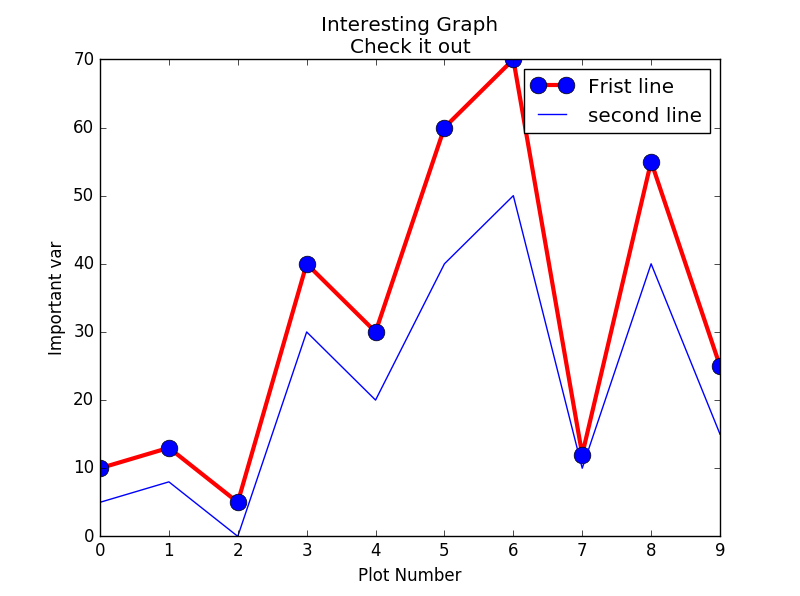条形图

import matplotlib.pyplot as plt
y1=[10,13,5,40,30,60,70,12,55,25]
x1=range(0,20,2)
x2=range(1,21,2)
y2=[5,8,0,30,20,40,50,10,40,15]
plt.bar(x1,y1,label='Frist line')
#plt.bar(x2,y2,label='second line',color='r')
plt.xlabel('Plot Number')
plt.ylabel('Important var')
plt.title('Interesting Graph\nCheck it out')
plt.legend()
plt.show()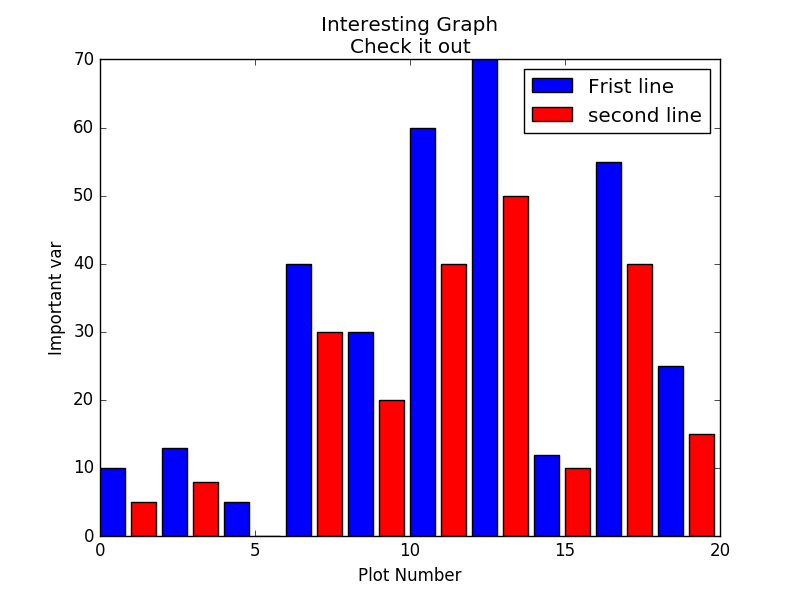直方图：

import matplotlib.pyplot as plt
population_ages = [22,55,62,45,21,22,34,42,42,4,99,102,
110,120,121,122,130,111,115,112,80,75,
65,54,44,43,42,48]
x=range(0,130,10)
plt.hist(population_ages,x,rwidth=0.8,color='r',histtype='stepfilled')

plt.xlabel('Plot Number')
plt.ylabel('Important var')
plt.title('Interesting Graph\nCheck it out')
plt.legend()
plt.show()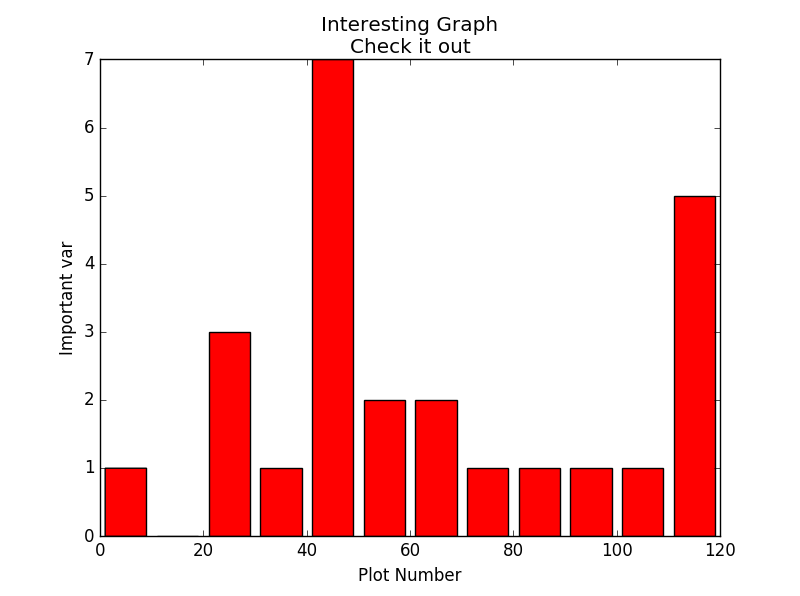散点图：

import matplotlib.pyplot as plt
population_ages = [22,55,62,45,21,22,34,42,42,4,99,102,
110,120,121,122,130,111,115,112,80,75,
65,54,44,43,42,48]
x=range(0,len(population_ages))
plt.scatter(x,population_ages,label='frist label',s=20)
help(plt.scatter)
plt.xlabel('x')
plt.ylabel('y')
plt.title('Interesting Graph\nCheck it out')
plt.legend()
plt.show()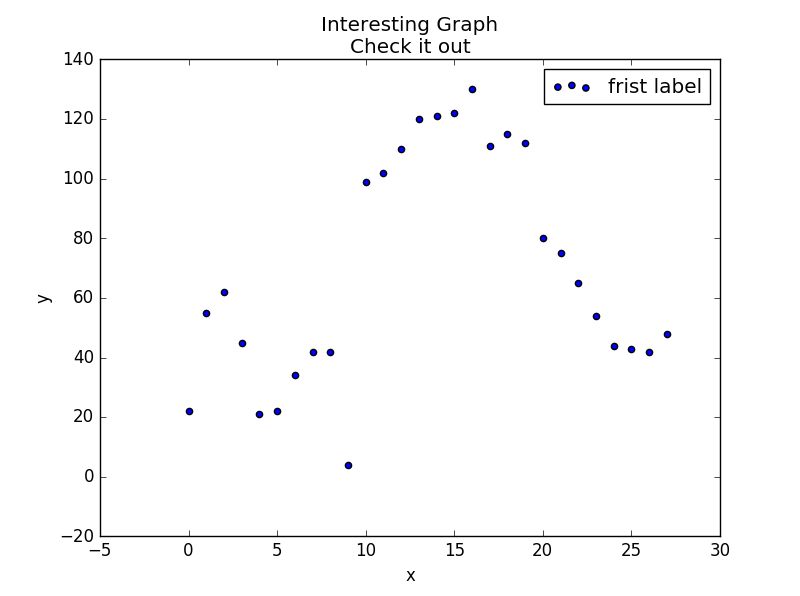展开全文折线图 python 条形图 散点图 直方图
• import matplotlib.pyplot as plt # figsize - 图像尺寸（figsize=(10,10)） # facecolor - 背景色（facecolor="blue"） # dpi - 分辨率（dpi=72） fig = plt.figure(figsize=(10,10),facecolor="blue") #figsize...
• 工具/原料pythonmatploblib方法/步骤1Matplotlib是一个python中的图像绘制库，先举一个简单的例子代码和绘制折线图的，如下图所示：2有时候需要在一个代码中绘制很多图像，需要创建多个figure对象，具体的代码和图像...

在论文写作中经常会出现多个算法效果比较的折线对比图，本文就介绍一下，怎样通过python中的matplotlib库来画出折线对比图。

工具/原料

python

matploblib

方法/步骤

1

Matplotlib是一个python中的图像绘制库，先举一个简单的例子代码和绘制折线图的，如下图所示：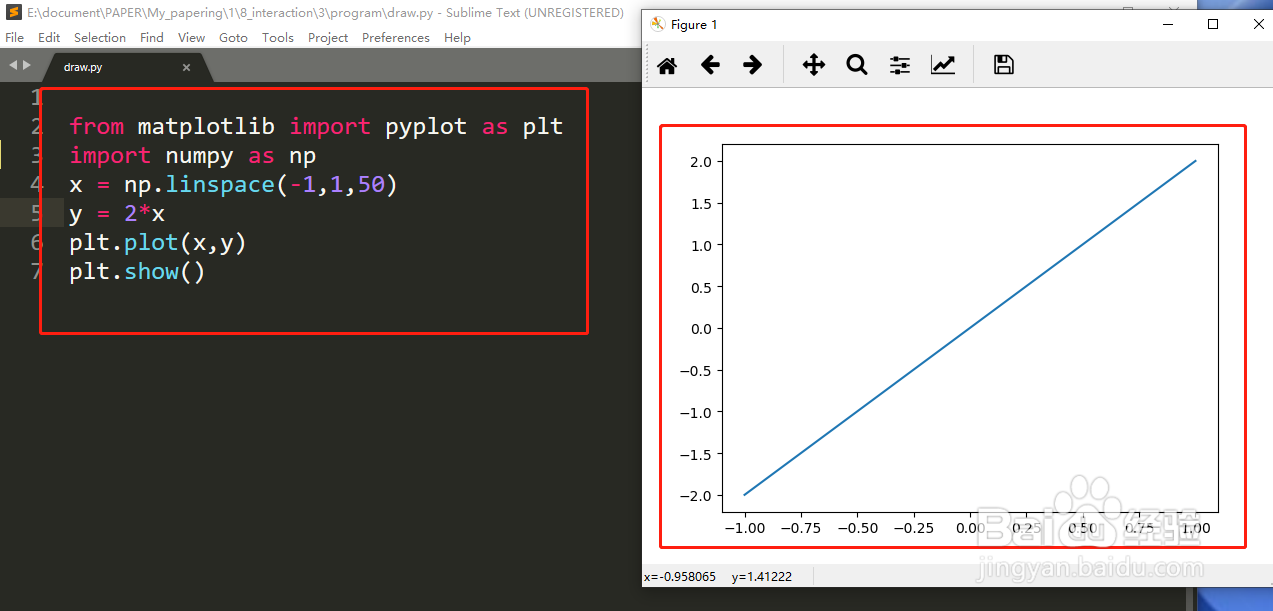2

有时候需要在一个代码中绘制很多图像，需要创建多个figure对象，具体的代码和图像如下图所示：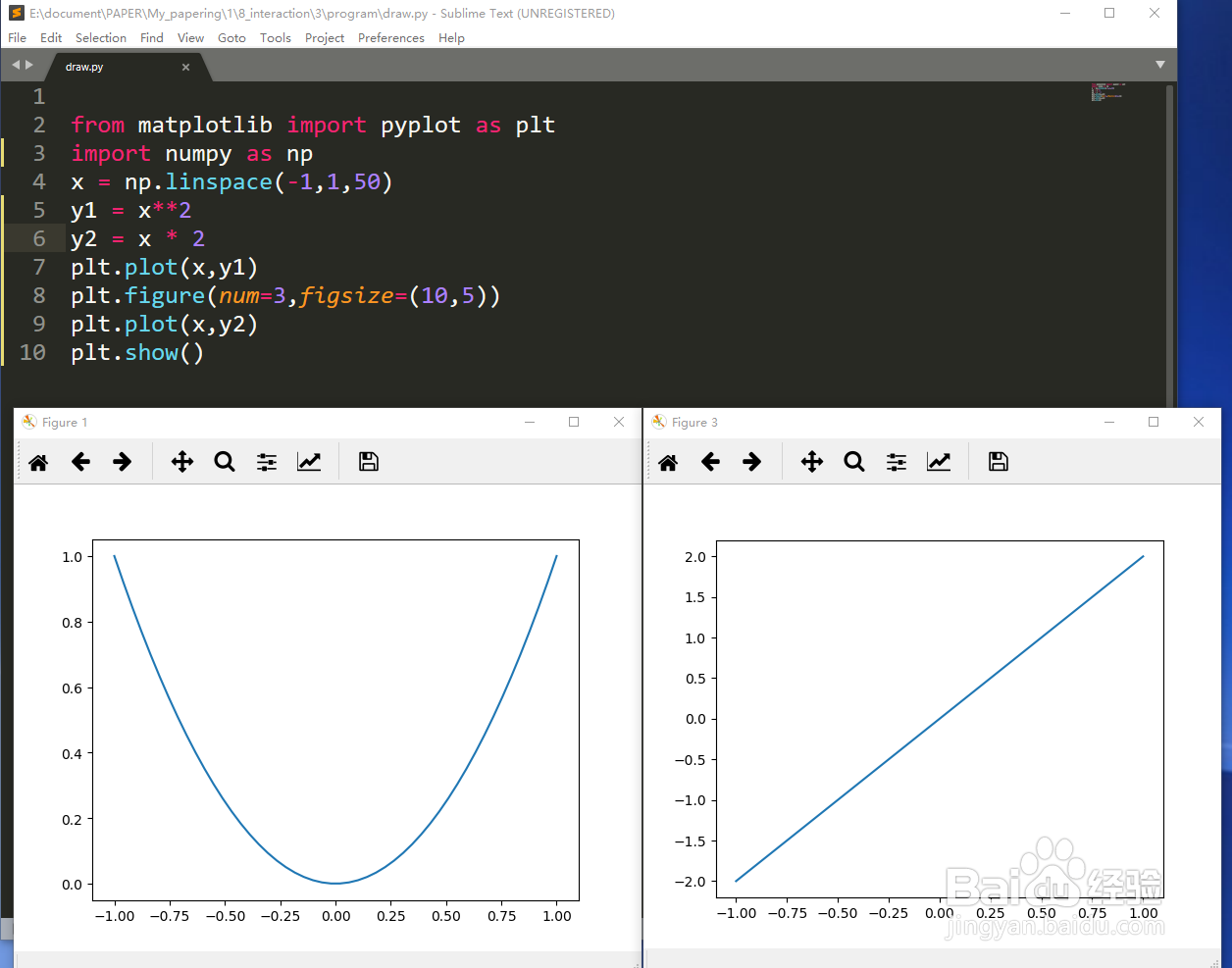3

如果想让图像改变颜色和样式，具体的代码和绘制图像所示：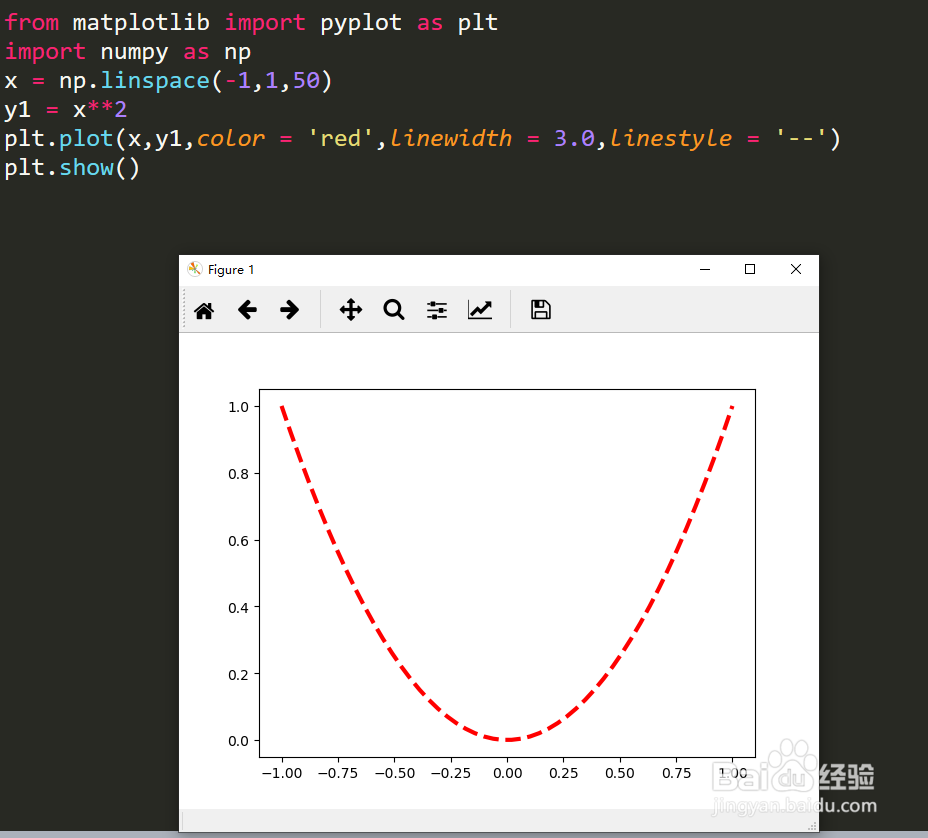4

如果要给图像设置横纵坐标轴，并在横纵坐标轴显示x，y的取值范围，具体的代码和绘制的图像如下所示：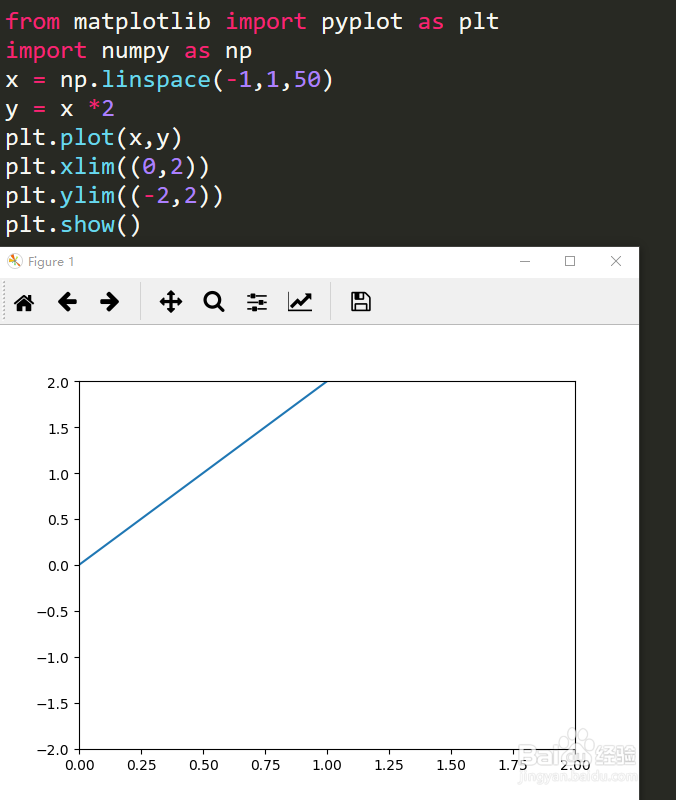5

如果要给图像设置横纵坐标轴起一个名字，具体的代码和绘制的图像如下所示：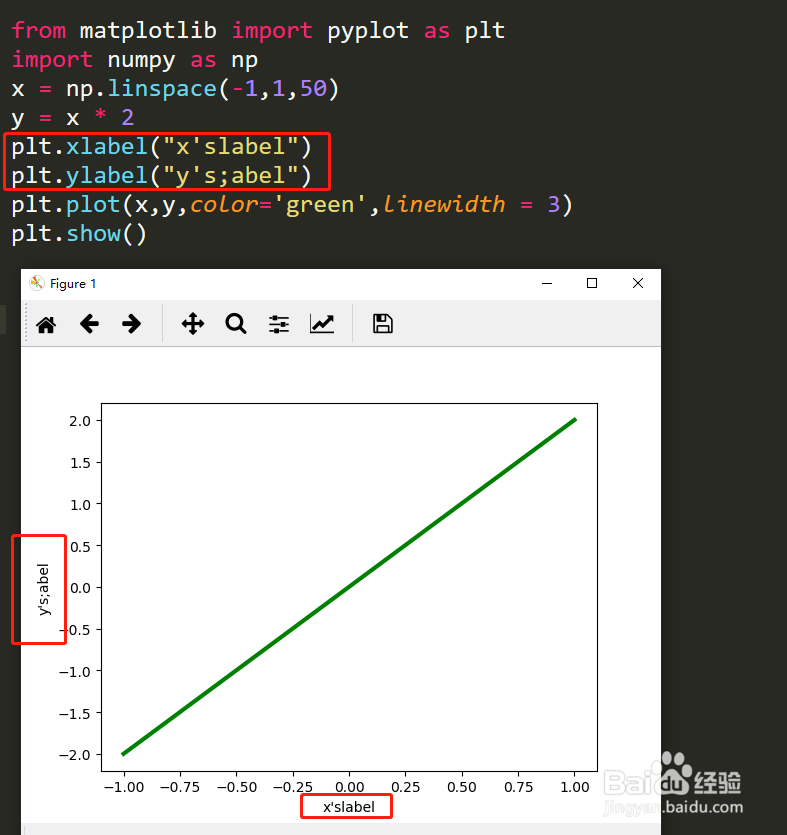6

如果把坐标轴换成不同的单位，则具体的代码和绘制的图像如下所示：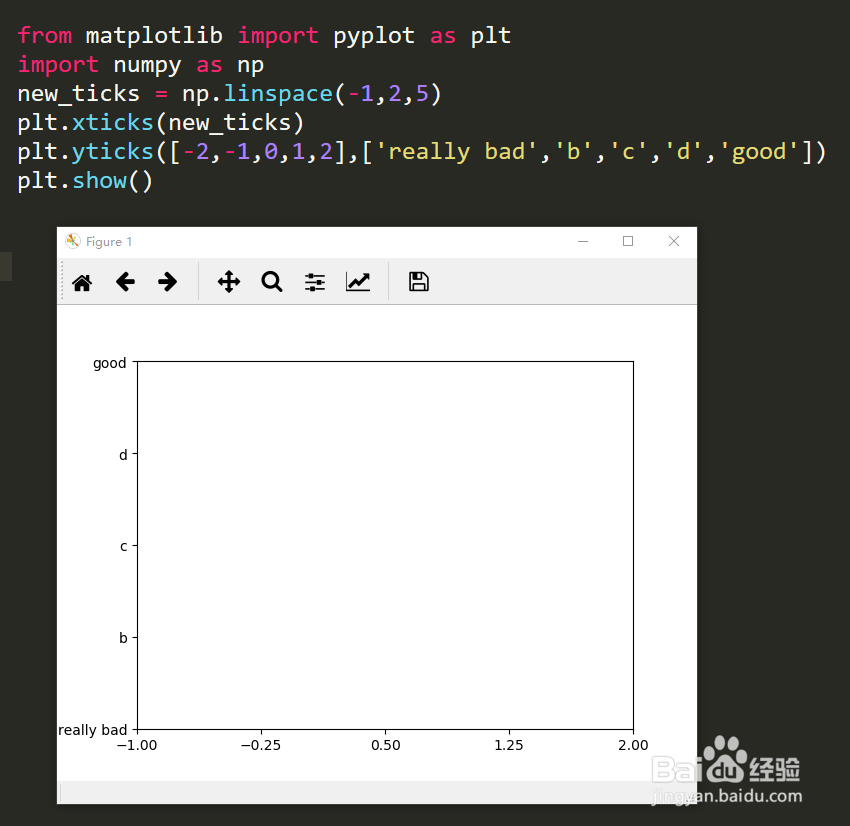7

如果给图像中加入图线的图标，则具体的代码和绘制的图像如下图所示：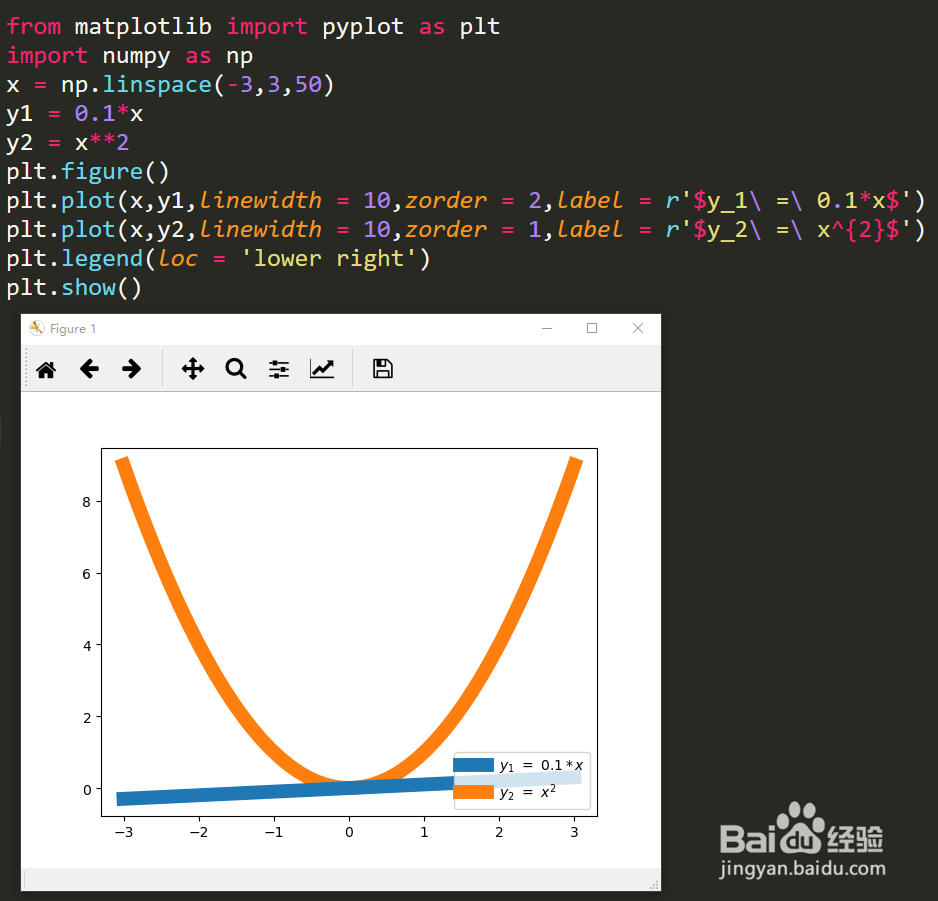8

如果要画散点图，具体的代码和绘制的图像如下图所示：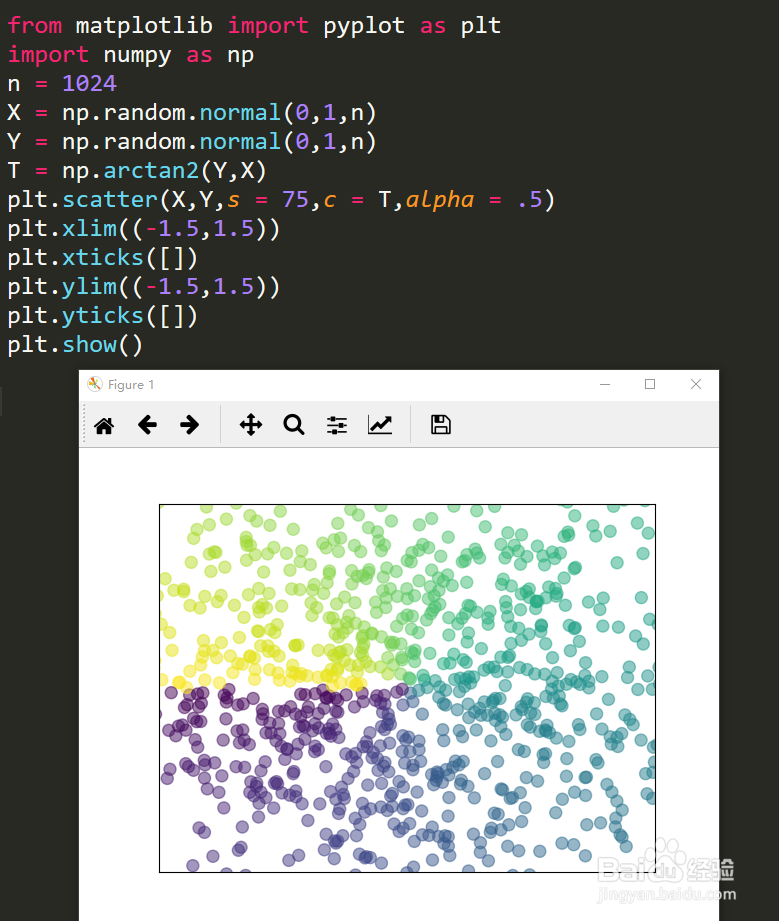END

注意事项

每次显示图片的时候需要用到plt.show()

经验内容仅供参考，如果您需解决具体问题(尤其法律、医学等领域)，建议您详细咨询相关领域专业人士。

举报作者声明：本篇经验系本人依照真实经历原创，未经许可，谢绝转载。

展开阅读全部

展开全文• import matplotlib.pyplot as plt x = np.linspace(0, 2 * np.pi, 10) y1, y2 = np.sin(x), np.cos(x) line1, =plt.plot(x, y1, marker='o', mec='r', mfc='w') line2, =plt.plot(x, y2, marker='*', ms=10) plt....
• 我整理了使用matplotlib绘制折线图的一般步骤，按照这个步骤走绘制折线图一般都没啥问题。其实用matplotlib库绘制折线图的过程，其实就是类似于数学上描点，连线绘制图形的过程。所有，这个过程就可以简单的规划为...python pycharm 开发语言
• matplotlib介绍 Matplotlib 是 Python 的绘图库。 它可与 NumPy 一起使用，提供了一种有效的 MatLab 开源替代方案。 它也可以和图形工具包一起使用，如 ...matplotlib绘制折线图 绘制一条折线的折线图 # -*- c...matplotlib 折线图 柱状图 python
• Python matplotlib绘制折线图

千次阅读 2020-11-01 22:10:31matplotlib 折线图
• 关闭网格线后，出正常，没有网格线。 ax1.grid(False)#网格线设置 ax2=ax1.twinx()#Y1，Y2共用一个X轴 ax2.grid(False) 打开Y轴的网格线后，明明没有打开X轴的网格线，X轴也出现了空白的网格线 各位这是怎么回事，...有问必答 python
• 使用 Python 的 matplotlib 库来画图还是比较方便的，今天整理一下 matplotlib 画折线图的方法。# coding:utf-8"""Author: roguesirDate: 2017/8/30GitHub: https://roguesir.github.comBlog: http:...
• matplotlib是Python中的一个第三方库。主要用于开发2D图表，以渐进式、交互式的方式实现数据可视化，可以更直观的呈现数据，使数据更具说服力。 一、安装matplotlib pip install matplotlib -i ...
• 使用matplotlib画折线图时可以如下方式指定线条颜色 import matplotlib.pyplot as plt plt.plot([1,2],[3.4],color="red") plt.show() 但是其实可以更简单的方法—字母来代替颜色 plt.plot([1,2],[3,4],'r'...matlab python 数据可视化
• Python实现画折线图的一个简单示例 # coding=utf-8 import numpy as np import matplotlib.pyplot as plt import matplotlib.font_manager as fm from scipy import interpolate font = 'TIMESBD.TTF' #字体文件，...python matplotlib 折线图
• matplotlib是python的一个模块，用于绘制各种图形，今天介绍一下折线图的绘制。 先举一个简单的例子： from matplotlib import pyplot as plt x=range(4,26,2) y=[32,43,21,54,32,3,21,32,21,32,43] plt.plot(x,y) ...python 数据分析
• import matplotlib.pyplot as plt import random 绘制基本图像 x = range(60) y_shanghai = [random.uniform(20, 50) for i in x] # 1.创建画布 plt.figure(figsize=(20, 8), dpi=100) # 2.绘制图像 plt.plot(x, y...python
• matplotlib是受MATLAB的启发构建的。...每天温度数据如下，[15, 12.2, 14, 14, 34, 43, 23, 34, 11.5, 22, 23, 12]，利用pyplot绘制折线图。 代码如下： from matplotlib import pyplot as plt x = range(2,
• 本文主要讲解如何使用Pyhon最流行的工具之一Matplotlib来绘制折线图： （一）安装Matplotlib使用Matplotlib来生成图表，为此需要使用pip包来对Matplotlib进行安装，执行命令如下： 为避免网络问题而导致下载并...python
• 记录下 使用python matplotlib从excel读取数据出来 反正就是各种拼凑 直接上结果代码 from datetime import date import pandas as pd import numpy as np import matplotlib.pyplot as plt import matplotlib as...python matplotlib
• 先上代码 import os ...import matplotlib.pyplot as plt with open('test1122/costTimemy.txt', 'r') as f: contents = f.readlines() print(len(contents)) moduletimes = [] totaltimes = [] ...python matplotlib 折线图
• csv数据如下所示： 代码如下所示： import pandas as pd import numpy as np import matplotlib.pyplot as plt plt.rcParams['font.sans-serif'] = 'simhei' plt.rcParams['axes.unicode_minus']=False data...python csv 可视化
• csv数据如下所示： 代码如下所示： import pandas as pd import numpy as np import matplotlib.pyplot as plt plt.rcParams['font.sans-serif'] = 'simhei' plt.rcParams['axes.unicode_minus']=False data...python csv 数据分析 可视化
• import matplotlib.pyplot as plt # 1.创建画布 # figsize:指定图的长宽 ...绘制折线图 plt.plot([1, 2, 3, 4, 5, 6 ,7], [17,17,18,15,11,11,13]) # 3.显示图像 plt.show() # 保存图片到指定路径 ...python opencv 可视化 matlab...